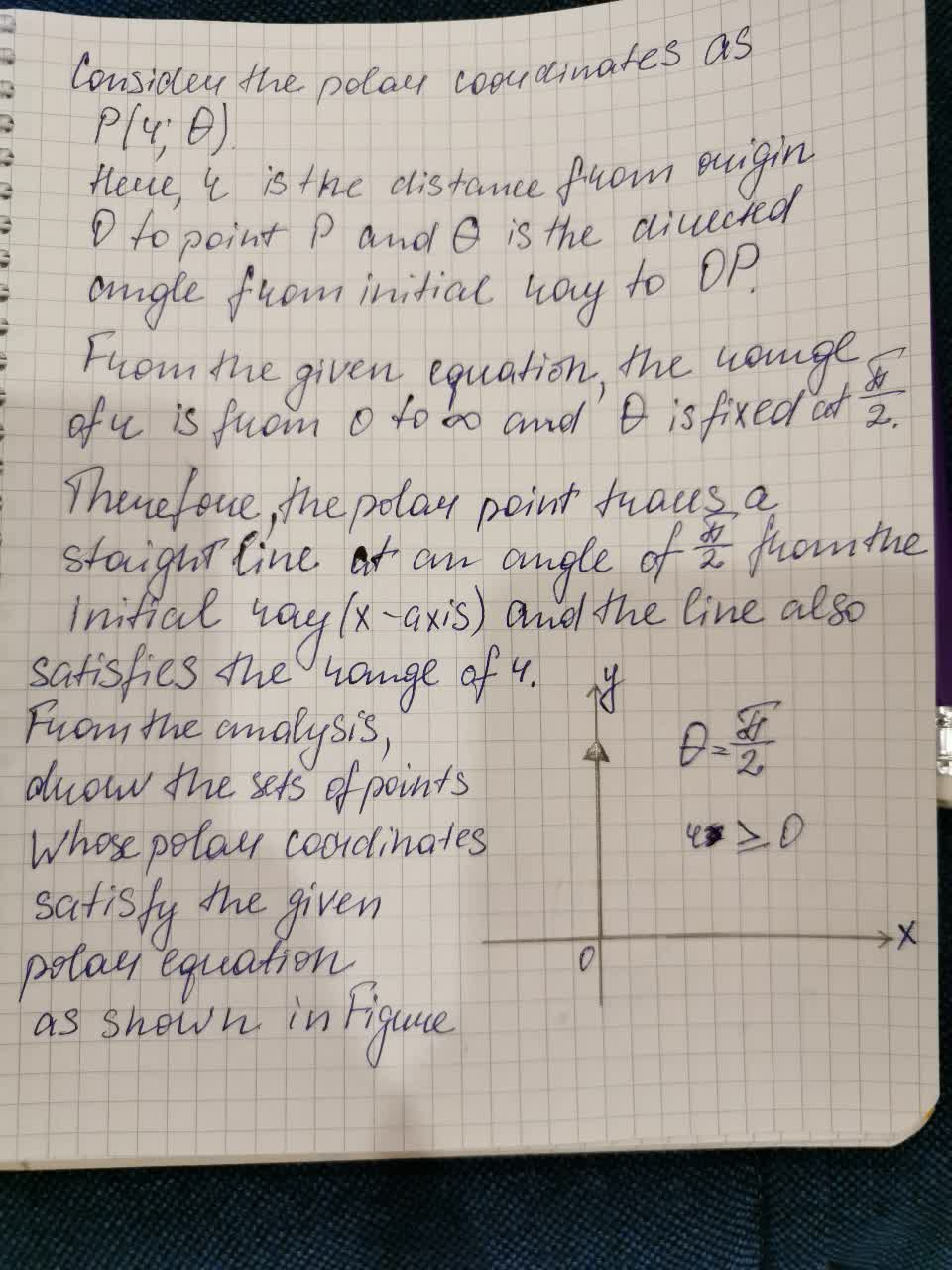# Graph the sets pf points whose polar coordinates satisfy the equations and inequalitiesTolnaio 2021-08-19 Answered
Graph the sets pf points whose polar coordinates satisfy the equations and inequalities $\theta =\frac{\pi }{2},r\ge 0$
You can still ask an expert for help

• Questions are typically answered in as fast as 30 minutes

Solve your problem for the price of one coffee

• Math expert for every subject
• Pay only if we can solve itpivonie8